Next: References Up: Boolean Functions Fitness Spaces Previous: Acknowledgements

# No Free Lunch Theorems (NFL)

Roughly speaking NFL says ``the average performance of [search] algorithms across all possible problems is identical'' [Wolpert and Macready1997, page 67,]. There are only 256 (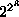) three input Boolean functions however it is not practical to use them to experimentally confirm any of the NFL theorems. This is because the number of fitness functions (i.e. problems) is too big.

In GP we consider only a small number of fitness functions, typically only one. The three input boolean functions are unusual in that 256 fitness functions have been investigated. However this is still a miniscule proportion of all the possible fitness functions and so NFL does not apply. Indeed the performance of three search algorithms (GP [Koza1992], hill climbing [Lang1995] and uniform random search) are not identical when averaged across all 256 three input Boolean functions.

Conventionally the fitness of a Boolean function of n inputs is defined as the number of times its output matches that required when tested upon all possible combinations of inputs. The total number of test patterns is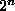. Therefore the fitness function yields a value in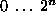and the total number of possible fitness functions is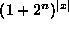where |x| is the size of the search space. If we consider searching in the space of trees then |x| is already very big and so considering allfitness functions is infeasible. (A program to calculate |x|

is available from ftp node ftp.mad-scientist.com directory pub/genetic-programming/code sub-directory gp-code in file ntrees.cc and via my home page).

If instead of considering searching the space of trees, we consider search in the space of theBoolean functions themselves then there are only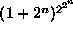possible fitness functions. In the case of n=3 this is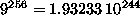. Clearly comparing the performance of two or more search algorithms on this number of problems is still infeasible.

If we limit ourselves to n=2, i.e. the 16 (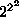) Boolean functions of 2 bits, there are only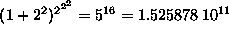possible fitness functions. While it might be feasible to evaluate the performance of various search algorithms on them and so experimentally confirm NFL, such a demonstration is unlikely to be persuasive due to the small size of the search space. The small number of points in the search space makes it likely that stochastic search techniques such as GAs will resample one or more points. NFL explicitly requires the algorithms not to do so. Any measured difference in performance between algorithms is likely to be due to this and stochastic noise inherent in randomised search techniques.Next: References Up: Boolean Functions Fitness Spaces Previous: Acknowledgements

William B Langdon
Tue Jun 16 15:05:48 BST 1998